Stick Diagrams UNITII Stick Diagrams 1 Stick Diagrams

• Slides: 57Stick Diagrams UNIT-II Stick Diagrams 1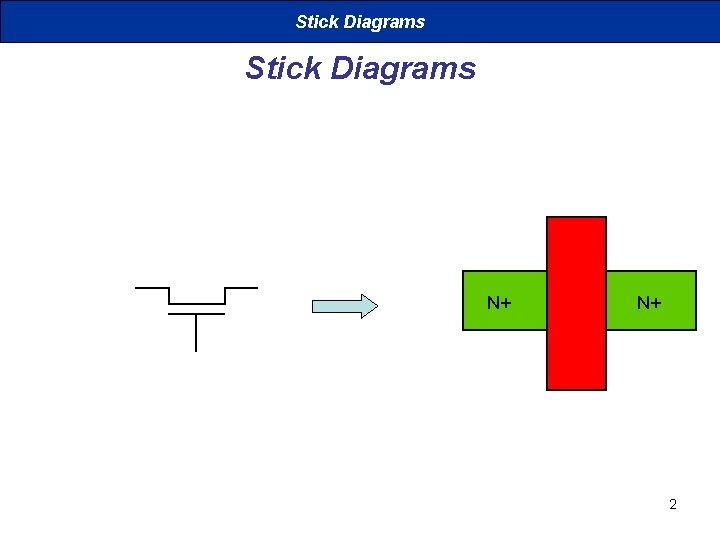Stick Diagrams N+ N+ 2Stick Diagrams VDD X X Stick Diagra m X X Gnd 3Stick Diagrams VDD X X Gnd 4Stick Diagrams § VLSI design aims to translate circuit concepts onto silicon. § stick diagrams are a means of capturing topography and layer information using simple diagrams. § Stick diagrams convey layer information through colour codes (or monochrome encoding). § Acts as an interface between symbolic circuit and the actual layout. 5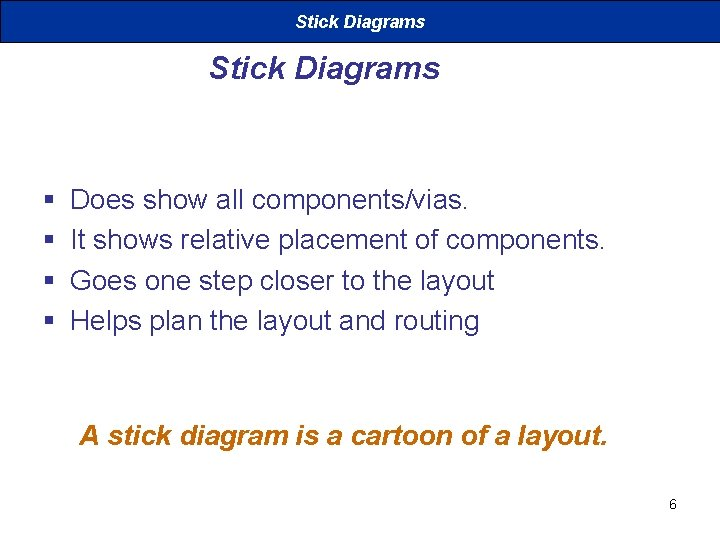Stick Diagrams § § Does show all components/vias. It shows relative placement of components. Goes one step closer to the layout Helps plan the layout and routing A stick diagram is a cartoon of a layout. 6Stick Diagrams § Does not show • • Exact placement of components Transistor sizes Wire lengths, wire widths, tub boundaries. Any other low level details such as parasitics. . 7Stick Diagrams – Notations Metal 1 poly ndiff pdiff Can also draw in shades of gray/line style. Similarly for contacts, via, tub etc. . 8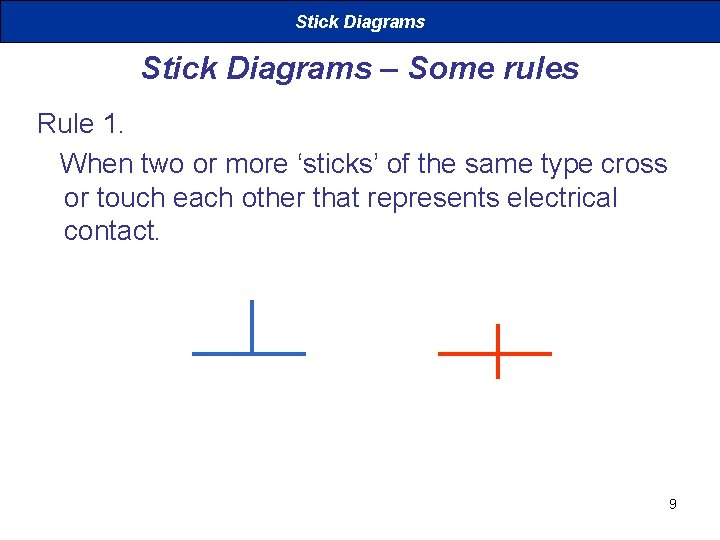Stick Diagrams – Some rules Rule 1. When two or more ‘sticks’ of the same type cross or touch each other that represents electrical contact. 9Stick Diagrams – Some rules Rule 2. When two or more ‘sticks’ of different type cross or touch each othere is no electrical contact. (If electrical contact is needed we have to show the connection explicitly). 10Stick Diagrams – Some rules Rule 3. When a poly crosses diffusion it represents a transistor. Note: If a contact is shown then it is not a transistor. 11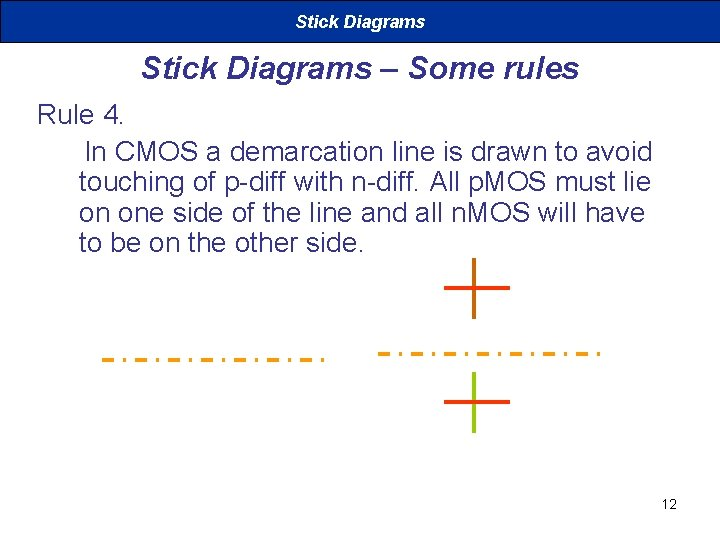Stick Diagrams – Some rules Rule 4. In CMOS a demarcation line is drawn to avoid touching of p-diff with n-diff. All p. MOS must lie on one side of the line and all n. MOS will have to be on the other side. 12Stick Diagrams How to draw Stick Diagrams 13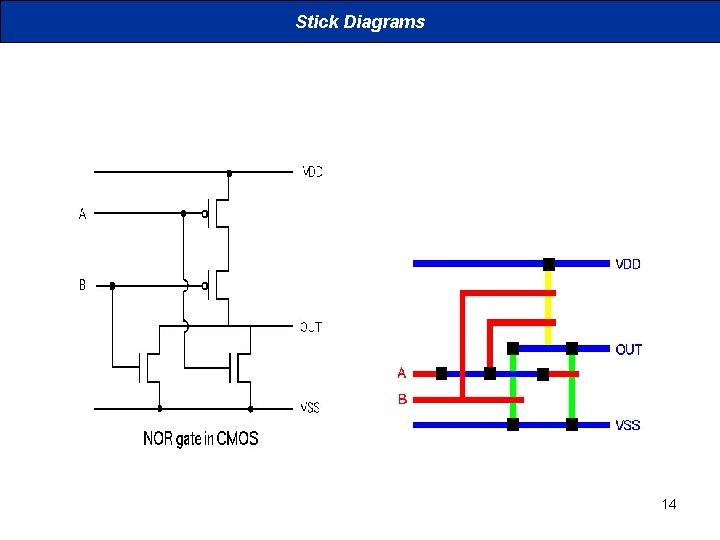Stick Diagrams 14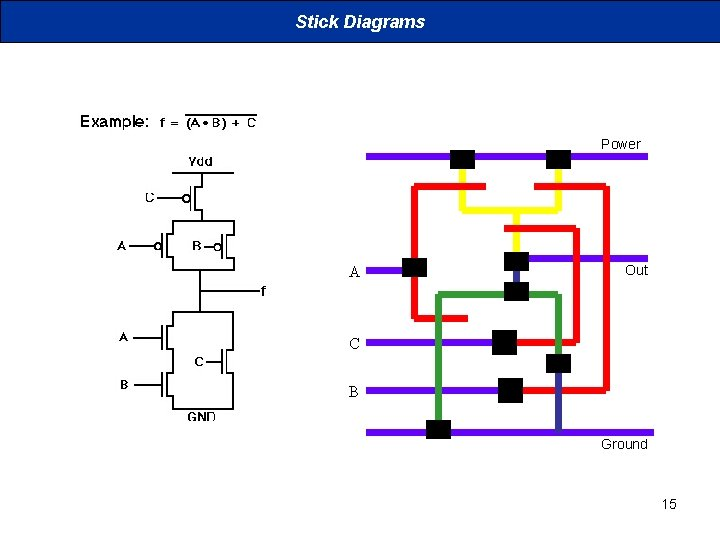Stick Diagrams Power A Out C B Ground 15Introduction to CMOS VLSI Design MOS devices: static and dynamic behavior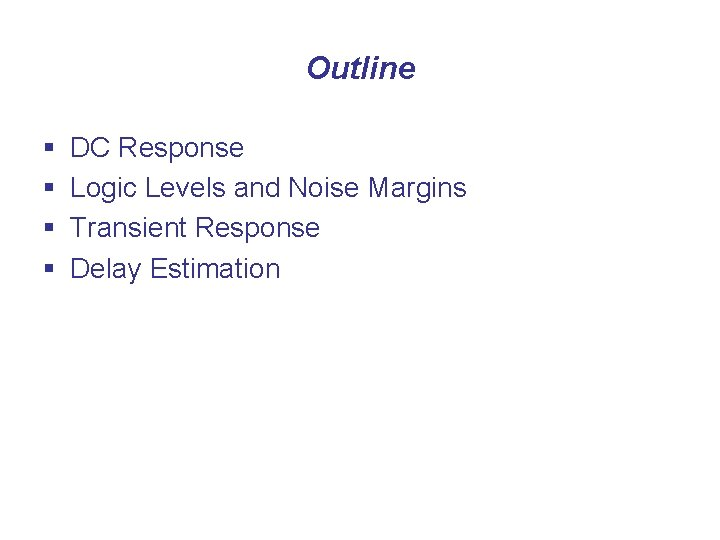Outline § § DC Response Logic Levels and Noise Margins Transient Response Delay EstimationDC Response § DC Response: Vout vs. Vin for a gate § Ex: Inverter • When Vin = 0 -> Vout = VDD • When Vin = VDD -> Vout = 0 • In between, Vout depends on transistor size and current • By KCL, must settle such that Idsn = |Idsp| • We could solve equations • But graphical solution gives more insight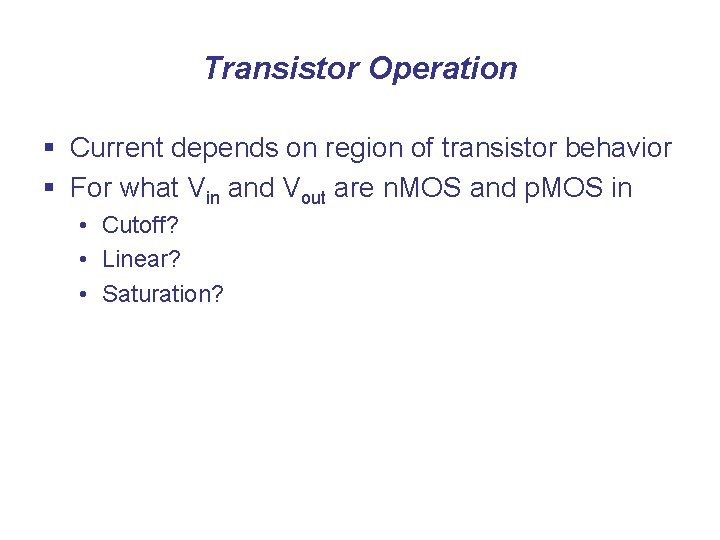Transistor Operation § Current depends on region of transistor behavior § For what Vin and Vout are n. MOS and p. MOS in • Cutoff? • Linear? • Saturation?n. MOS Operation Cutoff Vgsn < Linear Vgsn > Saturated Vgsn > Vdsn < Vdsn >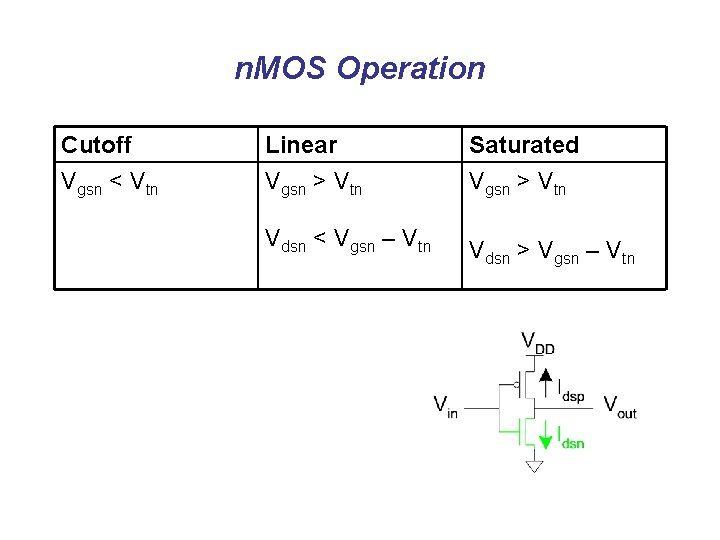n. MOS Operation Cutoff Vgsn < Vtn Linear Vgsn > Vtn Saturated Vgsn > Vtn Vdsn < Vgsn – Vtn Vdsn > Vgsn – Vtn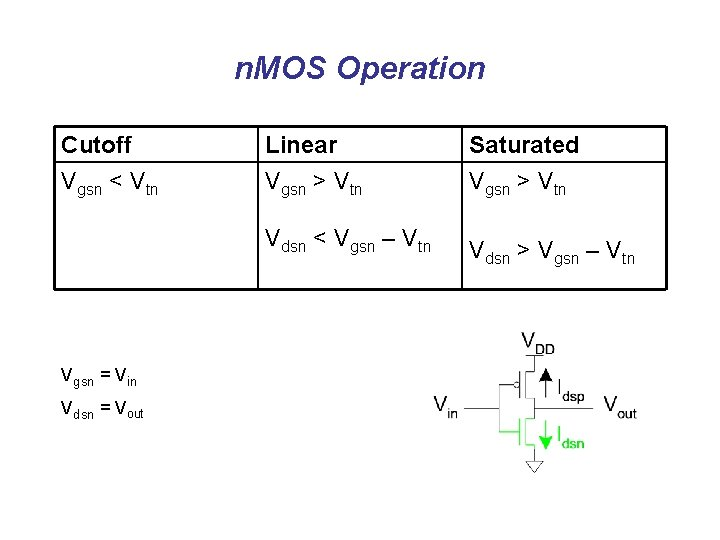n. MOS Operation Cutoff Vgsn < Vtn Vgsn = Vin Vdsn = Vout Linear Vgsn > Vtn Saturated Vgsn > Vtn Vdsn < Vgsn – Vtn Vdsn > Vgsn – Vtn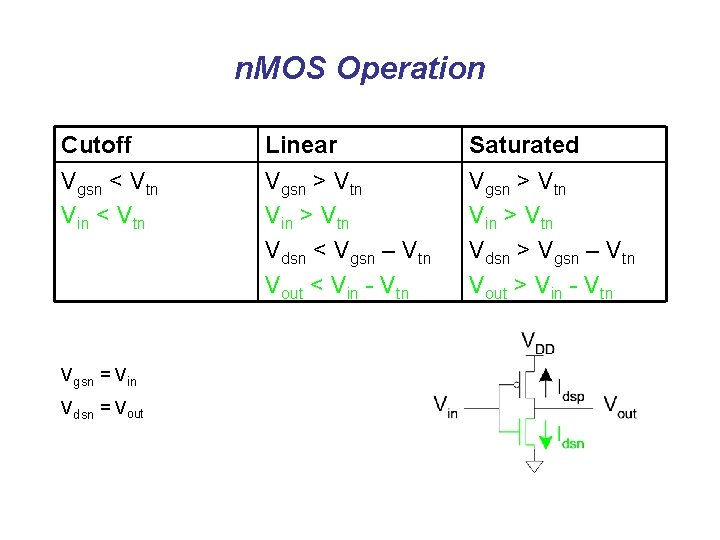n. MOS Operation Cutoff Vgsn < Vtn Vin < Vtn Vgsn = Vin Vdsn = Vout Linear Vgsn > Vtn Vin > Vtn Vdsn < Vgsn – Vtn Vout < Vin - Vtn Saturated Vgsn > Vtn Vin > Vtn Vdsn > Vgsn – Vtn Vout > Vin - Vtnp. MOS Operation Cutoff Vgsp > Linear Vgsp < Saturated Vgsp < Vdsp > Vdsp <p. MOS Operation Cutoff Vgsp > Vtp MOS equations Linear Vgsp < Vtp Saturated Vgsp < Vtp Vdsp > Vgsp – Vtp Vdsp < Vgsp – Vtp Slide 25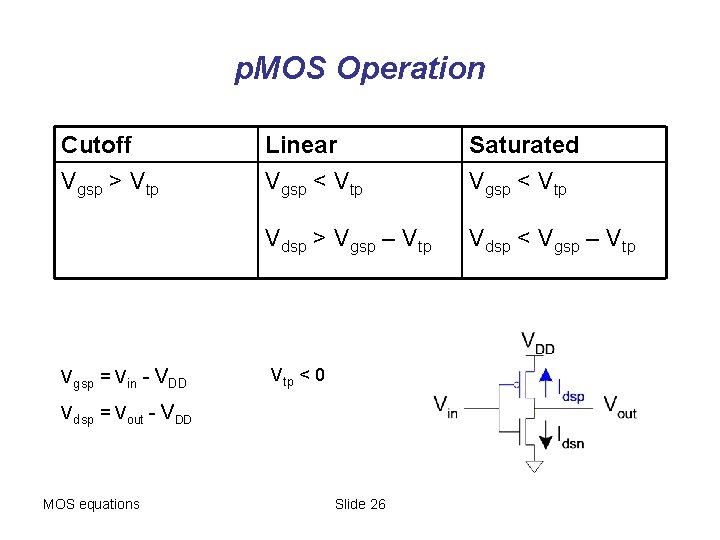p. MOS Operation Cutoff Vgsp > Vtp Vgsp = Vin - VDD Linear Vgsp < Vtp Saturated Vgsp < Vtp Vdsp > Vgsp – Vtp Vdsp < Vgsp – Vtp < 0 Vdsp = Vout - VDD MOS equations Slide 26p. MOS Operation Cutoff Vgsp > Vtp Vin > VDD + Vtp Vgsp = Vin - VDD Vdsp = Vout - VDD Linear Vgsp < Vtp Vin < VDD + Vtp Vdsp > Vgsp – Vtp Vout > Vin - Vtp < 0 Saturated Vgsp < Vtp Vin < VDD + Vtp Vdsp < Vgsp – Vtp Vout < Vin - Vtp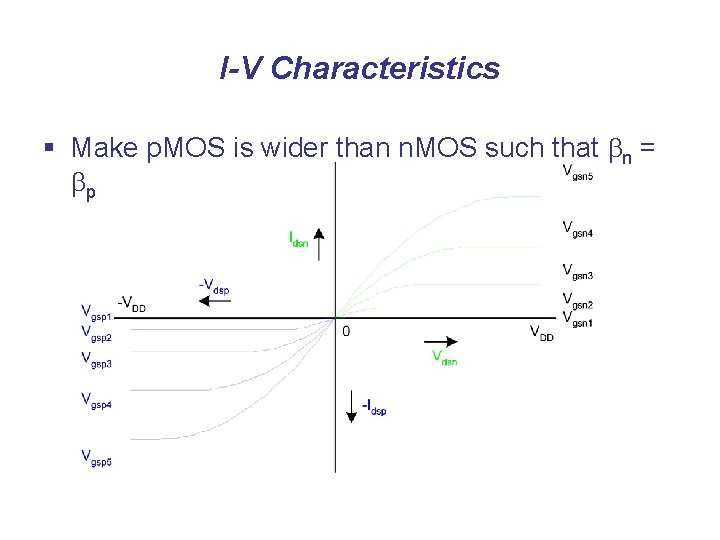I-V Characteristics § Make p. MOS is wider than n. MOS such that bn = bpCurrent vs. Vout, Vin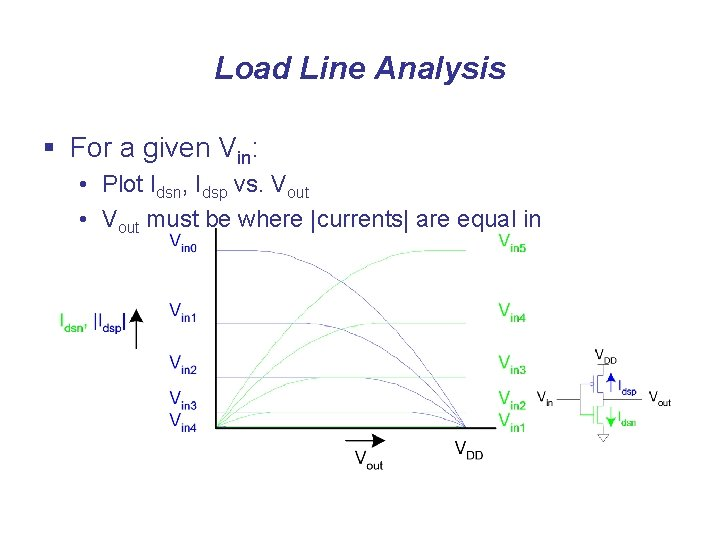Load Line Analysis § For a given Vin: • Plot Idsn, Idsp vs. Vout • Vout must be where |currents| are equal in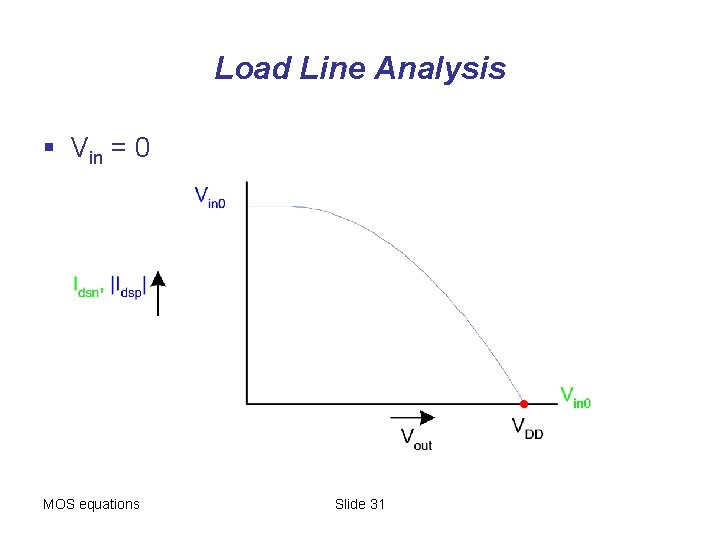Load Line Analysis § Vin = 0 MOS equations Slide 31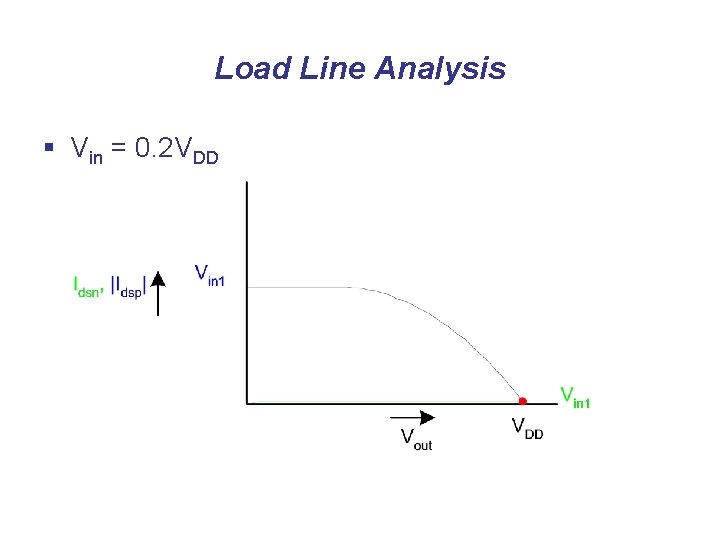Load Line Analysis § Vin = 0. 2 VDD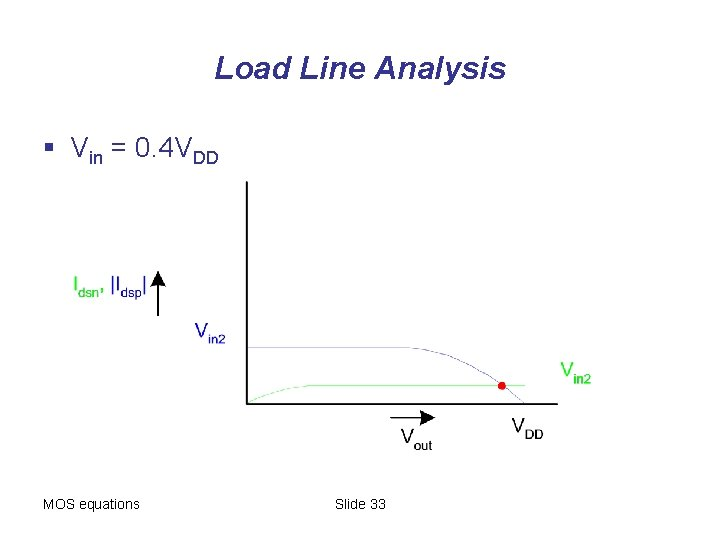Load Line Analysis § Vin = 0. 4 VDD MOS equations Slide 33Load Line Analysis § Vin = 0. 6 VDD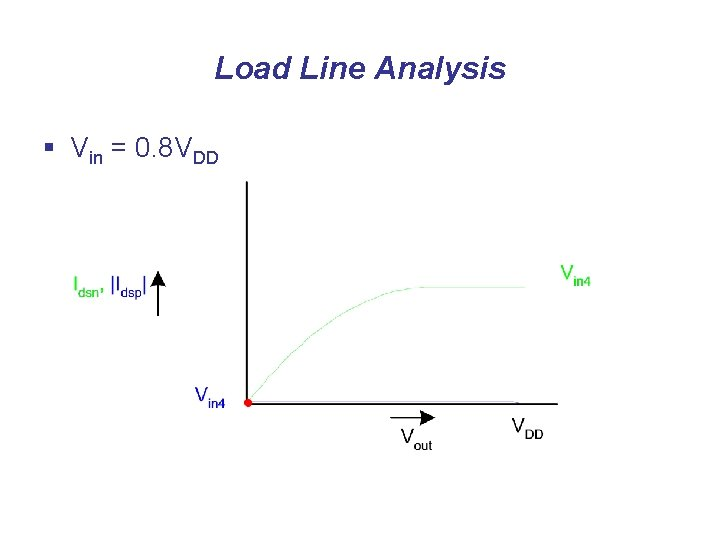Load Line Analysis § Vin = 0. 8 VDD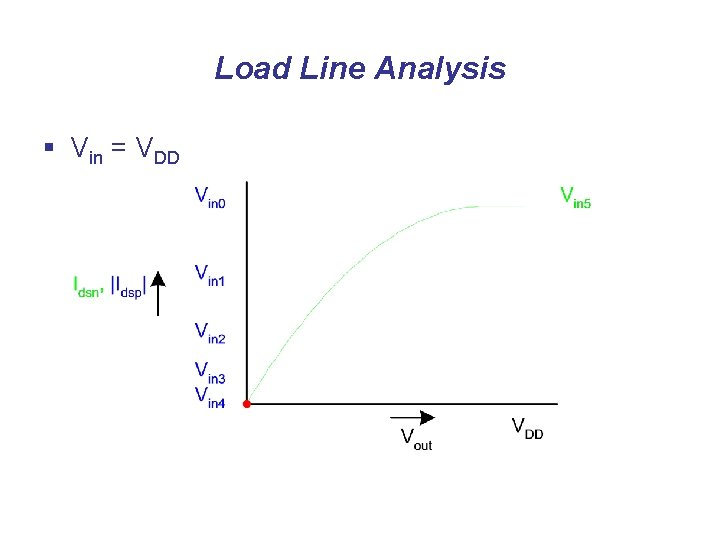Load Line Analysis § Vin = VDD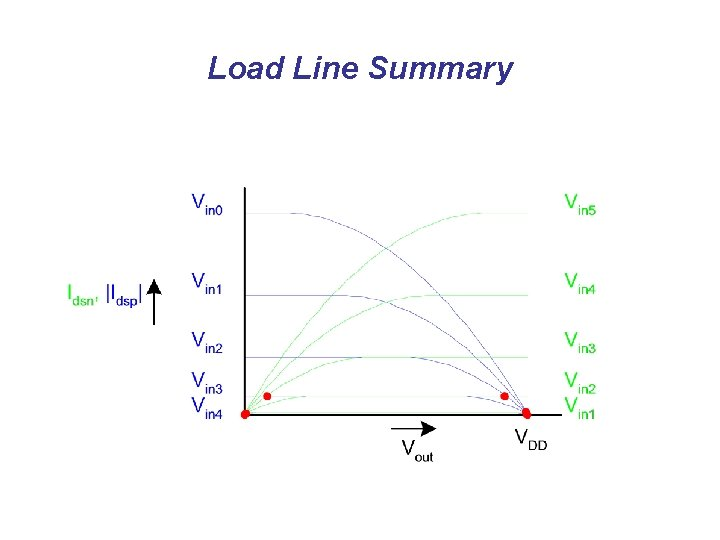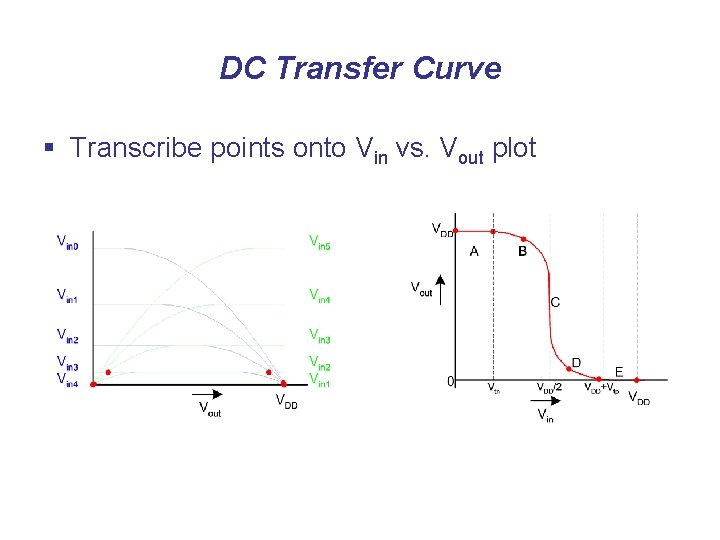DC Transfer Curve § Transcribe points onto Vin vs. Vout plotOperating Regions § Revisit transistor operating regions Region n. MOS p. MOS A Cutoff Linear B Saturation Linear C Saturation D Linear Saturation E Linear Cutoff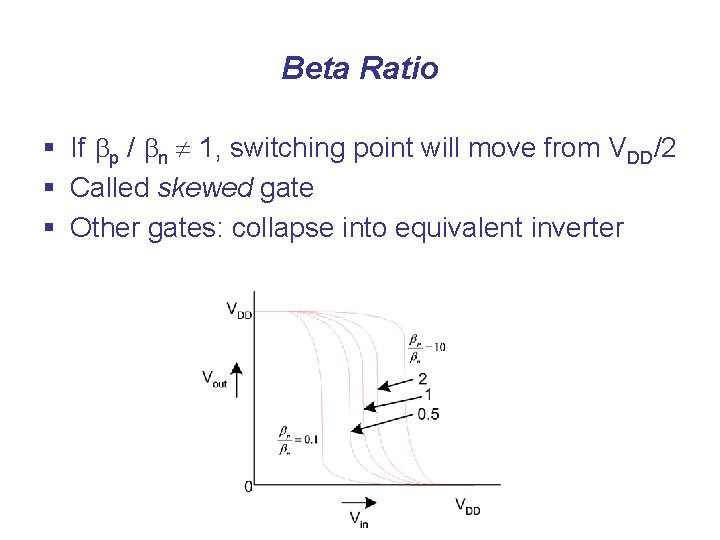Beta Ratio § If bp / bn 1, switching point will move from VDD/2 § Called skewed gate § Other gates: collapse into equivalent inverter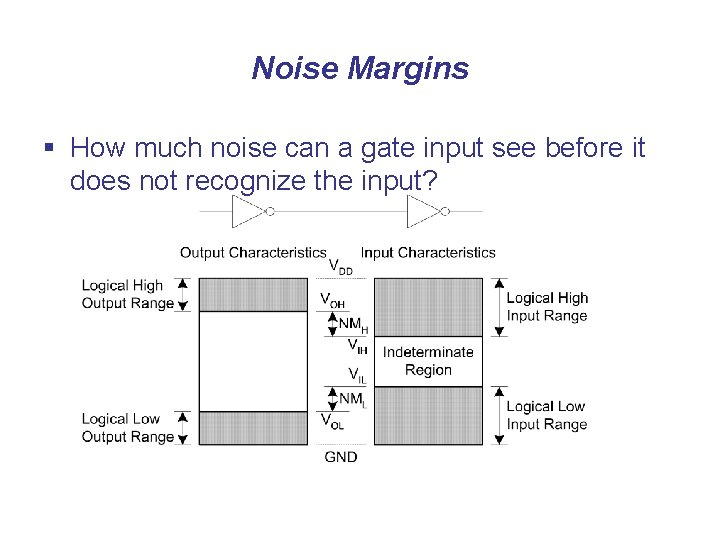Noise Margins § How much noise can a gate input see before it does not recognize the input?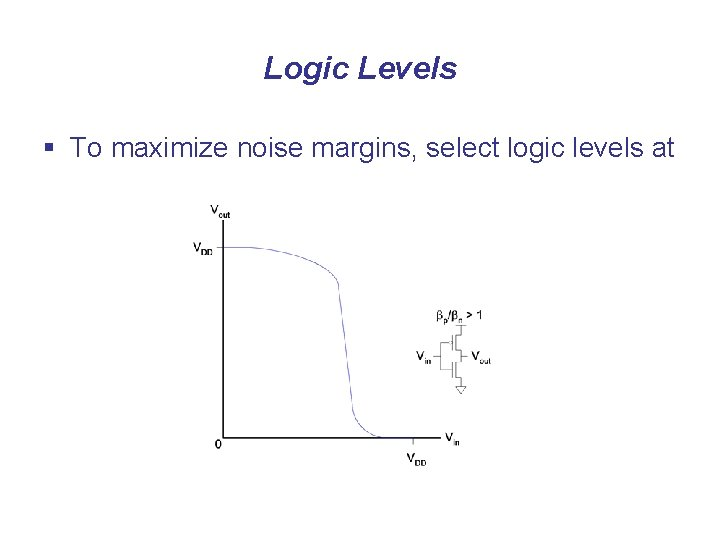Logic Levels § To maximize noise margins, select logic levels atLogic Levels § To maximize noise margins, select logic levels at • unity gain point of DC transfer characteristicTransient Response § DC analysis tells us Vout if Vin is constant § Transient analysis tells us Vout(t) if Vin(t) changes • Requires solving differential equations § Input is usually considered to be a step or ramp • From 0 to VDD or vice versaInverter Step Response § Ex: find step response of inverter driving load capInverter Step Response § Ex: find step response of inverter driving load capInverter Step Response § Ex: find step response of inverter driving load capInverter Step Response § Ex: find step response of inverter driving load cap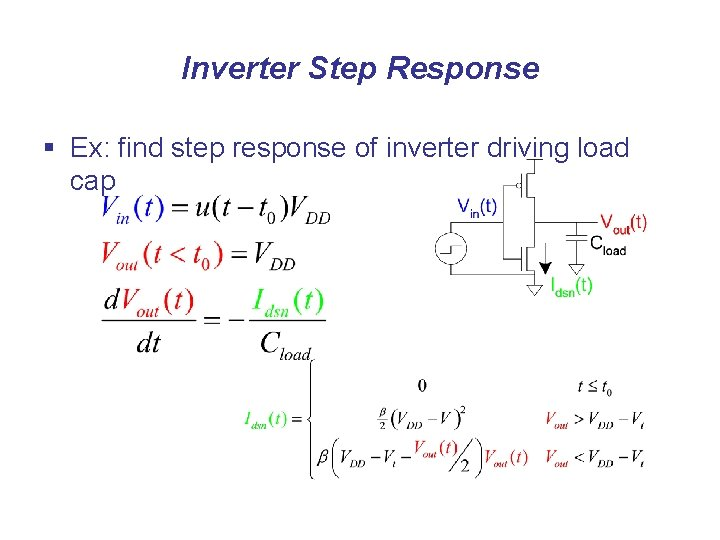Inverter Step Response § Ex: find step response of inverter driving load capInverter Step Response § Ex: find step response of inverter driving load cap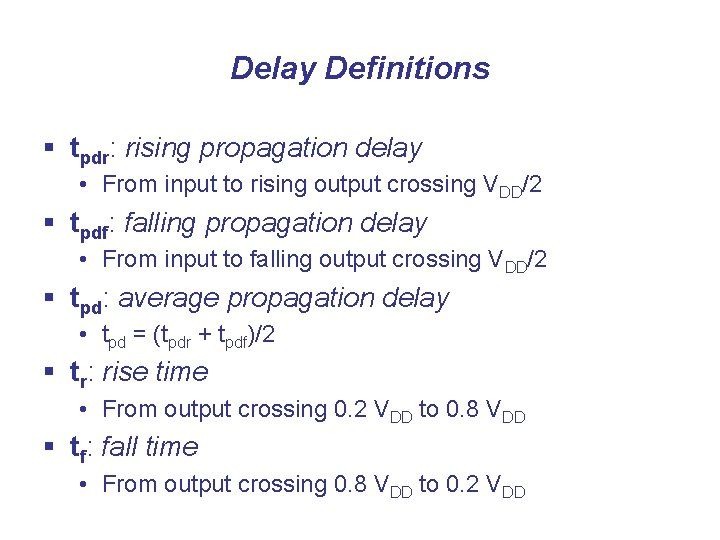Delay Definitions § tpdr: rising propagation delay • From input to rising output crossing VDD/2 § tpdf: falling propagation delay • From input to falling output crossing VDD/2 § tpd: average propagation delay • tpd = (tpdr + tpdf)/2 § tr: rise time • From output crossing 0. 2 VDD to 0. 8 VDD § tf: fall time • From output crossing 0. 8 VDD to 0. 2 VDD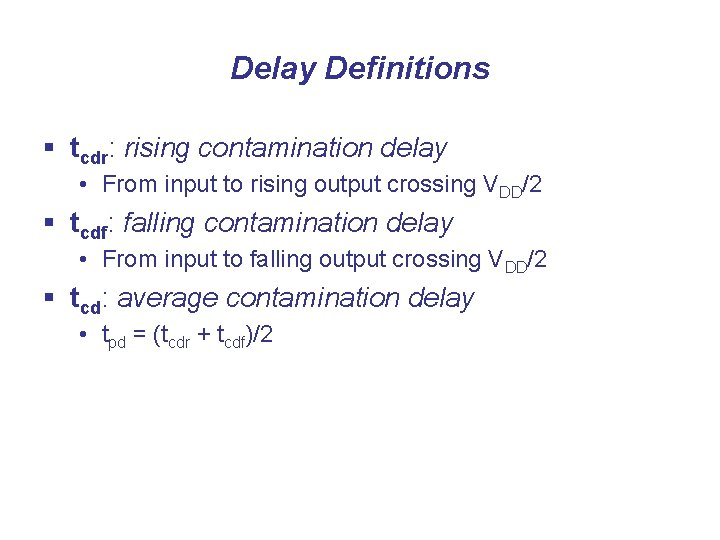Delay Definitions § tcdr: rising contamination delay • From input to rising output crossing VDD/2 § tcdf: falling contamination delay • From input to falling output crossing VDD/2 § tcd: average contamination delay • tpd = (tcdr + tcdf)/2Simulated Inverter Delay § Solving differential equations by hand is too hard § SPICE simulator solves the equations numerically • Uses more accurate I-V models too! § But simulations take time to writeDelay Estimation § We would like to be able to easily estimate delay • Not as accurate as simulation • But easier to ask “What if? ” § The step response usually looks like a 1 st order RC response with a decaying exponential. § Use RC delay models to estimate delay • C = total capacitance on output node • Use effective resistance R • So that tpd = RC § Characterize transistors by finding their effective R • Depends on average current as gate switchesRC Delay Models § Use equivalent circuits for MOS transistors • Ideal switch + capacitance and ON resistance • Unit n. MOS has resistance R, capacitance C • Unit p. MOS has resistance 2 R, capacitance C § Capacitance proportional to width § Resistance inversely proportional to width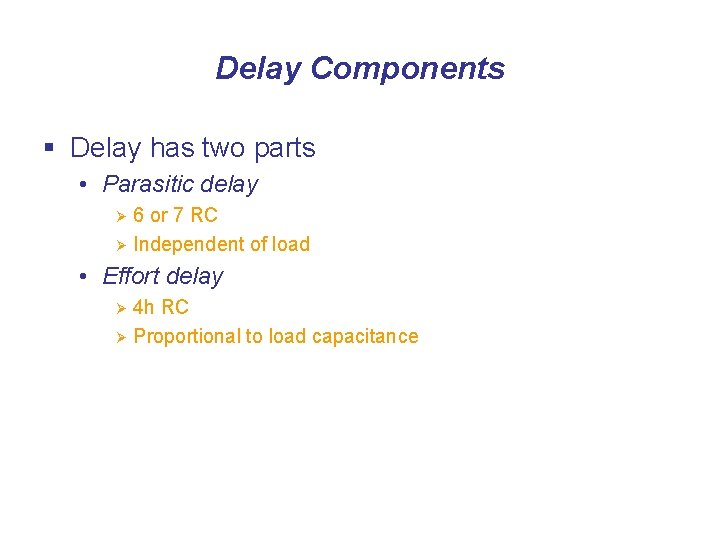Delay Components § Delay has two parts • Parasitic delay 6 or 7 RC Ø Independent of load Ø • Effort delay 4 h RC Ø Proportional to load capacitance ØDiffusion Capacitance § we assumed contacted diffusion on every s / d. § Good layout minimizes diffusion area § Ex: NAND 3 layout shares one diffusion contact • Reduces output capacitance by 2 C • Merged uncontacted diffusion might help too